## Modeling Planar Photonic Band Gap Structure

This example shows how to create and analyze microwave planar Photonic Band Gap (PBG) structures in Antenna Toolbox™. Photonic Band Gap structures consist of a periodic lattice which provides effective and flexible control of the Electromagnetic wave propagation in one or multiple directions. Microwave planar PBG structures were first introduced around the year 2000 by Prof. Itoh and his group. These structures create a stop band over a certain frequency range and are easy to implement by cutting periodic patterns on the metal ground plane.

### Design Frequency and System Parameters

The design shown in this example is the same as in . A 50-ohm conventional microstrip is designed on a RT/Duroid 6010 substrate, with dielectric constant of 10.5 and 25 mil thickness. The strip width is 27 mil. The period of the lattice on the back is kept at 200 mil. The overall PCB board size is 6 periods by 9 periods.

```period = 200*1e-3*0.0254; % period = 200mil; boardLength = period*6; boardWidth = period*9; boardThick = 25*1e-3*0.0254; % board is 25mil thick boardPlane = antenna.Rectangle('Length',boardLength,... 'Width',boardWidth); sub = dielectric('Name','Duroid6010','EpsilonR',10.5,... 'Thickness',boardThick); stripWidth = 27*1e-3*0.0254;% 27mil; stripLength = boardWidth; strip = antenna.Rectangle('Length',stripWidth,... 'Width',stripLength,'Center',[0,0]); ```

In our first study, a circle with radius of 25 mil is used as the unit etch shape on the ground. A lattice of size 3 by 9 are etched out from the ground plane. The constructed ground plane is shown below. Later, we also study performance of the microstrip with different circles with larger radius, radius equals to 50 mil and 90 mil.

```gnd = boardPlane; radius = 25*1e-3*0.0254; % hole radius = 25mil; posStart = [-period, -stripLength/2+period/2]; for i = 1:3 for j =1:9 pos = posStart+[(i-1)*period,(j-1)*period]; circle = antenna.Circle('Radius',radius,... 'Center',pos,'NumPoints',16); gnd = gnd - circle; end end figure; show(gnd); axis equal; ```Here we combine the top microstrip, substrate and etched ground plane into pcbStack object for meshing and full wave analysis. The final constructed geometry is shown below:

```obj = pcbStack('Name','2D Bandgap Structure'); obj.BoardShape = boardPlane; obj.BoardThickness = boardThick; obj.Layers = {strip,sub,gnd}; obj.FeedLocations = [0,-boardWidth/2,1,3;0,boardWidth/2,1,3]; obj.FeedDiameter = stripWidth/2; figure; show(obj); axis equal; title(obj.Name); ```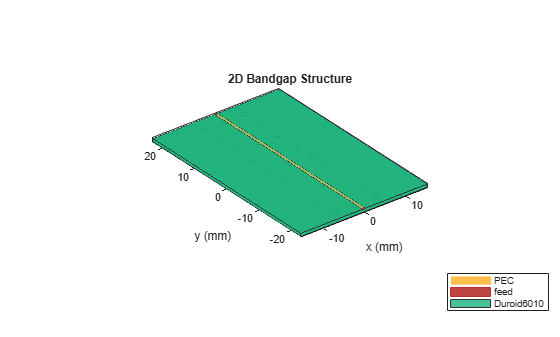We manually mesh the structure using manual mesh mode in the toolbox to better control the output triangles and tetrahedra.

```figure; mesh(obj,'MaxEdgeLength',12*stripWidth,... 'MinEdgeLength',stripWidth,'GrowthRate',0.85); ```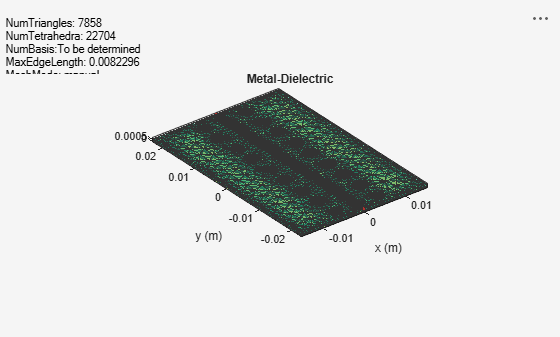In order to observe the band gap effect, we compute the S-parameters for the 2-port system. The band gap effect is shown in the S21 parameter. In the analysis, we calculated the S-parameters from 2 GHz to 16 GHz, and plot the S21 and S11 for the three different circle radii. The results for all s-parameter analysis has been precomputed and stored in a MAT-file.

`freq= linspace(2e9,16e9,141);`

`sparam = sparameters(obj,freq);`

`figure;`

`rfplot(sparam,1,1,'-o');`

`hold on;`

`rfplot(sparam,2,1,'--o');`

```load('atx_bandgap_data.mat','sparam_25mil'); load('atx_bandgap_data.mat','sparam_50mil'); load('atx_bandgap_data.mat','sparam_90mil'); figure; subplot(3,1,1); rfplot(sparam_25mil,1,1,'-'); text(4e9,-40,'Radius = 25 mil', 'FontSize',8, 'Color', 'm') hold on; rfplot(sparam_25mil,2,1,'--'); legend off; subplot(3,1,2); rfplot(sparam_50mil,1,1,'-'); text(4e9,-40,'Radius = 50 mil', 'FontSize',8, 'Color', 'm') hold on; rfplot(sparam_50mil,2,1,'--'); legend off; subplot(3,1,3); rfplot(sparam_90mil,1,1,'-'); text(4e9,-40,'Radius= 90 mil', 'FontSize',8, 'Color', 'm') hold on; rfplot(sparam_90mil,2,1,'--'); ```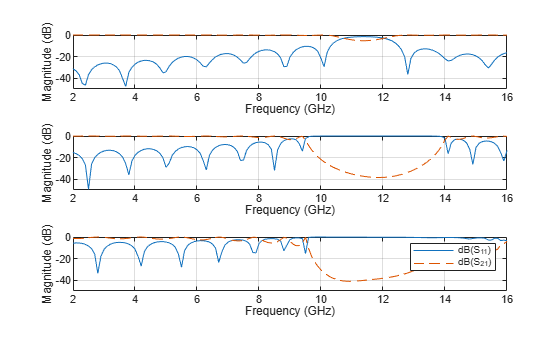From the calculated s-parameters, it is clearly seen that there is a stop band around 11GHz, which converts the 50-ohm matched transmission line into a bandstop filter. By varying the etching shape on the groundplane, different filter structures such as low pass or high pass, etc. can be realized.

### 90-degree Bend Microstrip Structure

As shown below, a compensated right-angle microstrip bend with patterned groundplane is created . The etched circles on the ground plane follow the right-angle bend.

```bendboardLength = period*9; bendboardWidth = period*9; boardThick = 25*1e-3*0.0254; % board is 25mil thick bendboardPlane = antenna.Rectangle('Length',bendboardLength,'Width',bendboardWidth); bendgnd = bendboardPlane; stripLength = bendboardWidth/2; strip_1 = antenna.Rectangle('Length',stripLength,'Width',stripWidth,'Center',[stripLength/2,0]); strip_2 = antenna.Rectangle('Length',stripWidth,'Width',stripLength+stripWidth/2,'Center',[0,-stripLength/2+stripWidth/4]); bendstrip = strip_1+strip_2; radius = 50*1e-3*0.0254; % hole radius = 50mil; posStart = [-period, -stripLength+period/2]; pos = zeros(27,2); for i = 1:3 for j =1:6 pos = posStart+[(i-1)*period,(j-1)*period]; circle = antenna.Circle('Radius',radius,'Center',pos,'NumPoints',15); bendgnd = bendgnd - circle; end end posStart = [period, -period]; for i = 1:3 for j = 1:3 pos = posStart+[(i)*period,(j-1)*period]; circle = antenna.Circle('Radius',radius,'Center',pos); bendgnd = bendgnd - circle; end end figure; show(bendgnd); axis equal; ```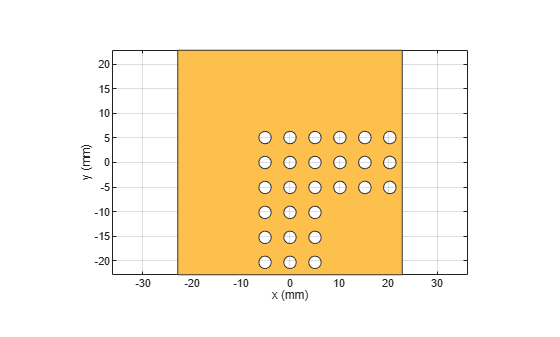### PCB Stack Creation

Create the layer stack up by organizing the PCB layers, setting the feed locations for the ports.

```bendobj = pcbStack('Name','2D Bandgap Bend Structure'); bendobj.BoardShape = bendboardPlane; bendobj.BoardThickness = boardThick; bendobj.Layers = {bendstrip,sub,bendgnd}; bendobj.FeedLocations = [0,-bendboardLength/2,1,3;bendboardWidth/2,0,1,3]; bendobj.FeedDiameter = stripWidth/2; figure; show(bendobj); axis equal; title(bendobj.Name); ```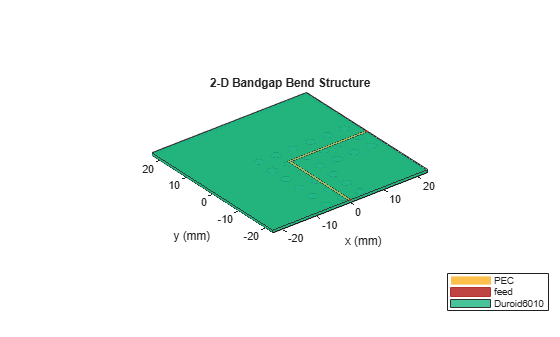The S-parameters for the structure is calculated. The analysis results compare favorably with the measured results reported in and the PBG properties of the structure are captured effectively. It is noted here that the analysis predicts almost perfect reflection within the stop band while the measured results shown in Fig. 3 of  reveal the presence of a loss mechanism that improves the impedance match slightly.

`freq= linspace(2e9,16e9,141);`

`sparam = sparameters(obj,freq);`

`figure;`

`rfplot(sparam,1,1,'-o');`

`hold on;`

`rfplot(sparam,2,1,'--o');`

```load('atx_bandgap_data.mat','sparam_bend_50mil'); figure; rfplot(sparam_bend_50mil,1,1,'-'); hold on; rfplot(sparam_bend_50mil,2,1,'--'); ```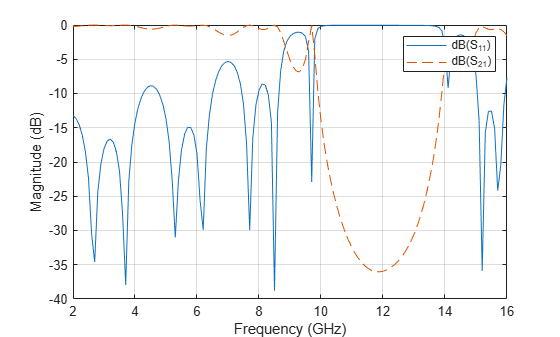### Conclusion

The result obtained for the three designs matches well with the result published in .

### Reference

 V. Radisic, Y. Qiang, R. Coccioli, and T. Itoh, "Novel 2-D Photonic Bandgap Structure for Microstrip Lines", IEEE Microwave and Guided Wave Letters, vol. 8, No. 2, 1998;